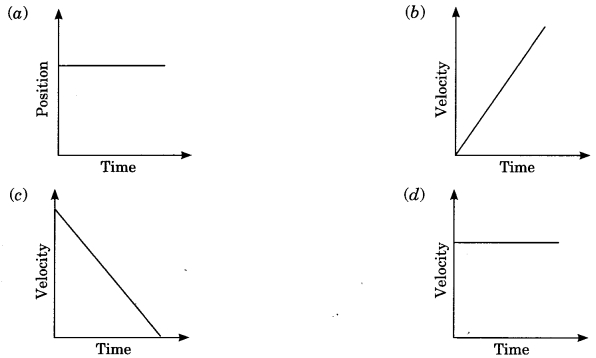# CBSEtips.in

## Saturday, 20 February 2021

### CBSE Class 9 Science - MCQ and Online Tests - Physics - Unit 1 - Motion

#### CBSE Class 9 Science – MCQ and Online Tests – Physics – Unit 1 – Motion

Every year CBSE  schools conducts Annual Assessment exams for 6,7,8,9,11th standards. These exams are very competitive to all the students. So our website provides online tests for all the 6,7,8,9,11th standard’s subjects. These tests are also very effective and useful for those who preparing for any competitive exams like Olympiad etc. It can boost their preparation level and confidence level by attempting these chapter wise online tests.

These online tests are based on latest CBSE syllabus. While attempting these our students can identify the weak lessons and continuously practice those lessons for attaining high marks. It also helps to revise the NCERT textbooks thoroughly.

#### CBSE Class 9 Science – MCQ and Online Tests – Physics – Unit 1 – Motion

Question 1.
Slope of a velocity-time graph gives
(a) the distance
(b) the displacement
(c) the acceleration
(d) the speed

Answer: (c) the acceleration

Question 2.
If the displacement of an object is proportional to square of time, then the object moves with
(a) uniform velocity
(b) uniform acceleration
(c) increasing acceleration
(d) decreasing acceleration

Answer: (b) uniform acceleration

Question 3.
Suppose a boy is enjoying a ride on a merry-go-round which is moving with a constant speed of 10 ms-1 It implies that the boy is
(a) at rest
(b) moving with no acceleration
(c) in accelerated motion
(d) moving with uniform velocity

Answer: (c) in accelerated motion

Question 4.
Area under a ? -1 graph represents a physical quantity which has the unit
(?) m2
(b) m
(c) m3
(d) ms-1

Question 5.
Which of the following situations is possible?
(a) An object can have acceleration, but constant velocity.
(b) The velocity of an object may be zero but acceleration is not zero.
(c) Distance and the magnitude of displacement are equal in circular motion.
(d) Average speed and the magnitude of average velocity are always equal in circular motion.

Answer: (b) The velocity of an object may be zero but acceleration is not zero.

Question 6.
Which of the following figures correctly represents uniform motion of a moving object?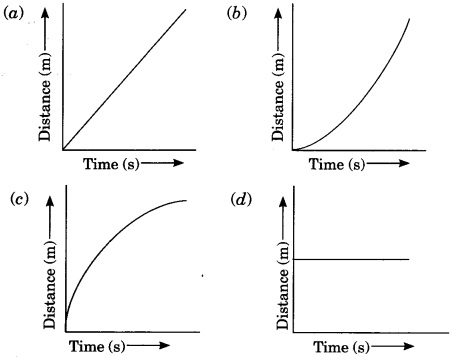Question 7.
Which of the following graphs is not possible?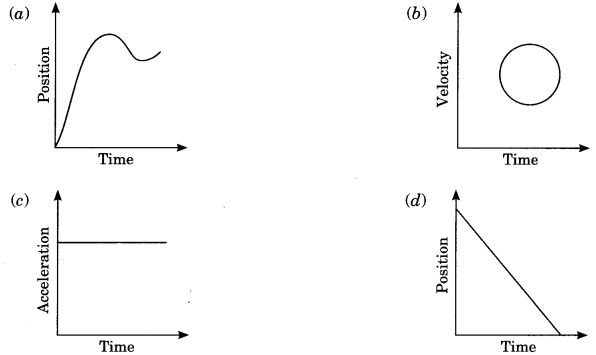Question 8.
A particle is moving in a circular path of radius r. The displacement after half a circle would be:
(a) Zero
(b) pr
(c) 2r
(d) 2pr

Question 9.
In which of the following cases of motions, the distance moved and the magnitude of displacement are equal?
(a) If the car is moving on a straight road
(b) If the car is moving in Circular path
(c) The pendulum is moving to and fro
(d) The earth is revolving around the sun.

Answer: (a) If the car is moving on a straight road

Question 10.
A boy goes from A to B with a velocity of 20 m/min and comes back from B to A with a velocity of 30 m/min. The average velocity of the boy during the whole journey is
(a) 24 m/min
(b) 25 m/s
(c) Zero
(d) 20 m/min

Answer: (a) 24 m/min

Question 11.
Velocity-time graph of an object is given below. The object has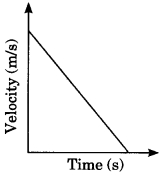(a) Uniform velocity
(b) Uniform speed
(c) Uniform retardation
(d) Variable acceleration

Answer: (c) Uniform retardation

Question 12.
A body is projected vertically upward from the ground. Taking vertical upward direction as positive and point of projection as origin, the sign of displacement of the body from the origin when it is at height h during upward and downward journey will be
(a) Positive, positive
(b) Positive, negative
(c) Negative, negative
(d) Negative, positive

Answer: (a) Positive, positive

Question 13.
According to the given velocity-time graph, the object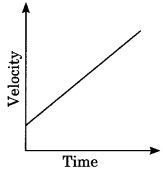(a) is moving with uniform velocity
(b) has some initial velocity
(c) is moving uniformly with some initial velocity
(d) is at rest

Answer: (b) has some initial velocity

Question 14.
The numerical ratio of displacement to distance for a moving object is
(a) always less than 1
(b) always equal to 1
(c) always more than 1
(d) equal or less than 1

Answer: (d) equal or less than 1

Question 15.
The ratio of speed to the magnitude of velocity when the body is moving in one direction is
(a) Less than one
(b) Greater than one
(c) Equal to one
(d) Greater than or equal to one

Answer: (c) Equal to one

Question 16.
A body is thrown vertically upward with velocity u, the greatest height h to which it will rise is,
(a) ulg
(b) u2l2g
(c) u2lg
(d) ul2g

Question 17.
A car is moving along a straight road with uniform velocity. It is shown in the graph.